# Varistor | Working Principle | Types | Metal Oxide Varistor

### Varistor:

Varistor is used to protect the semiconductor against over voltage. At the same time, it plays major role in high voltage transmission in electrical, it protects the high voltage equipment from lighting over voltage and surge voltage. It is two terminals, solid state semiconductor device.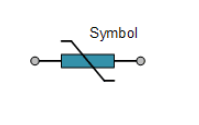### What is Varistor (Varistor Working Principle):

Varistor is nothing but a variable resistor. From variable => vari + resistor => stor = varistor. They are not like ohmic resistor such as variable resistor or potentiometer; they are non-ohmic resistor.  Ohmic resistor => the resistor should obey ohms’ law, Non-ohmic resistor => the resistor does not obey ohms’ law. It other words they are called as Non-liners resistor or voltage dependent resistor VDR.

The main difference between resistor and VDR is that the resistor’s resistance can be varied only with the manual action but the resistance of the varistor can be varied by varying the applied voltage. The operation of varistor is similar to the PN diode for reverse bias operation.

Types of Varistor:

• Metal oxide varistor
• Silicon carbide varistor

For this we take MOV metal-oxide varistor which is the most common type of varistor. This type contains a ceramic mass of zinc oxide grains, in a matrix of other metal oxides (such as small amounts of bismuth, cobalt, manganese) sandwiched between two metal plates (the electrodes). The boundary between each grain and its neighbour forms a diode junction, Just like a series connected diodes. Under normal condition, the Varistor’s junction does not conduct current, but when we increase the voltage beyond the reverse breakdown voltage, the diode junction starts conducts the current.

### Physical appearance of Varistor: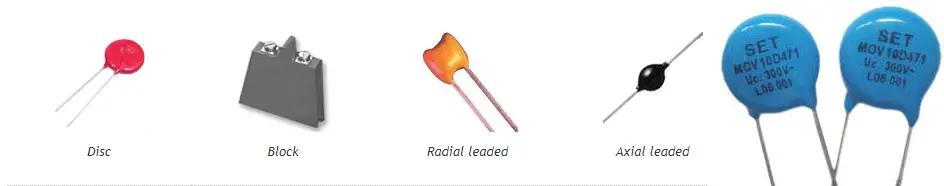Actually, varistor and ceramic capacitor seems similarly in an electronics circuit. But functionally, capacitor does not protect the circuit against transient surges, but varistor does. One of the most common sources of voltage transients are inductive loads such as Induction Motors, transformer magnetizing currents, DC motor switching applications and surges from the switching-on of fluorescent lighting circuits or other supply surges; they produce the transient voltage V is equal to L(di/dt).

### Electrical Characteristics of Varistor:

Let we take varistor static resistance: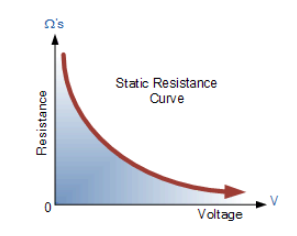See the graph of varistor, which resistance is decreasing as the voltage increases, but as per ohms’ law the V-I characteristics of a constant resistor is always straight line. Therefore, the current flow is directly proportional to the potential difference between the resistor.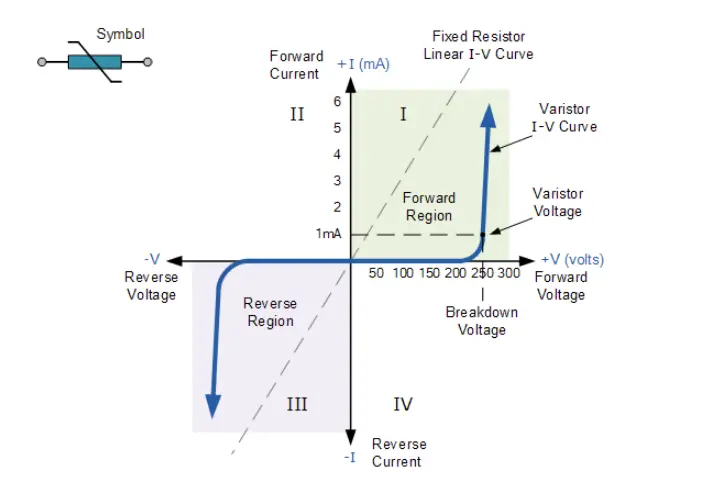But Coming to the varistor, The V-I characteristics is not a straight-line, For Some instance (0-200Volts) the varistor offers high resistance typically open circuit, Since the net current flow through the varistor is zero. Further When We increasing the Voltage across the varistor (200 to 250 Volts), then it starts conducting very few micro amperes, it is negligible.

At a point of 250 Volts the varistor allows 1mA. This is also called as rated voltage or clamping voltage of the varistor. The manufacturers are generally rating a varistor by this value. If the applied voltage is more than the rated voltage the Varistor allows large amount of current for increasing a small amount of voltage. At the same time the transient voltage is reduced below the rated voltage, then the varistor increases the resistance.

### Capacitance Effect in varistor:

As we know a varistor is generally connected in between a higher potential to lower potential and conducting region is acts a dielectric medium. These arrangement acts a parallel capacitance.  Every semiconductor varistor has a capacitance value that depends directly on its area and varies inversely with its thickness.

In DC, varistor does not affect by the capacitance effect. Since capacitor acts as pure open circuit (Xc= maximum) for the DC source. At the same time, when we increase the applied voltage more than clamping voltage, the varistor do the normal operation.

In AC circuit, The capacitive reactance is depending upon the applied source frequency (Xc=1/2πfC). Capacitive Current Ic= Vapplied/Xc. Since the increasing the frequency of the source, causes increases the leakage current. Therefore, while designing a varistor for AC circuits, the frequency effect must be taken care.

### Application of Varistor: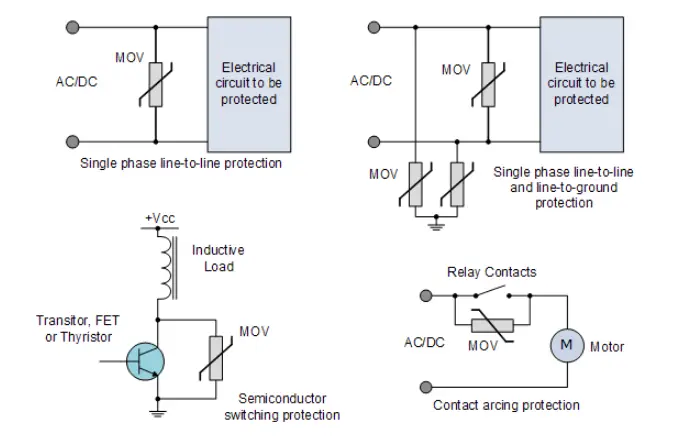• Power Supply protection
• VFD
• Transient Voltage Surge Suppressor TVSS
• Electronics Equipment Protection
• Transmission Line